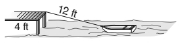Chapter 11.1, Problem 32E### Elementary Geometry for College St...

6th Edition
Daniel C. Alexander + 1 other
ISBN: 9781285195698

#### Solutions

Chapter
Section### Elementary Geometry for College St...

6th Edition
Daniel C. Alexander + 1 other
ISBN: 9781285195698
Textbook Problem
3 views

# In Exercises 27 to 34, use the drawings, where provided, to solve each problem. Angle measures should be given to the nearest degree; distances should be given to the nearest tenth of a unit.A 12-ft rope secures a rowboat to a pier that is 4ft above the water. Assume that the lower end of the rope is at “water level”. What is the angle formed by the rope and the water? Assume that the rope is taut.To determine

To find:

The angle formed by the water and the rope.

Explanation

In a right angle triangle,

1) The side PQ, which is opposite to the right angle PRQ is called the hypotenuse.

(The hypotenuse is the longest side of the right triangle.)

2) The side RQ is called the adjacent side of angle θ.

3) The side PR is called the opposite side of angle θ.

Hence, the sine ratio for an acute angle is oppositehypotenuse

Given:

From, the given diagram,

Opposite side = 4 ft and hypotenuse = 15 ft

Let α be the angle formed by the water and the rope.

We know that,

sinθ=oppositeHyp

### Still sussing out bartleby?

Check out a sample textbook solution.

See a sample solution

#### The Solution to Your Study Problems

Bartleby provides explanations to thousands of textbook problems written by our experts, many with advanced degrees!

Get Started

#### Show that f is continuous on ( , ). f(x)={1x2ifx1lnxifx1

Single Variable Calculus: Early Transcendentals, Volume I

#### List all of the subsets of {a, b, c, d}.

Mathematical Excursions (MindTap Course List)

#### Let and g(x) = x + 3. Then (g ∘ h)(x) = ______.

Study Guide for Stewart's Single Variable Calculus: Early Transcendentals, 8th

#### Find each value of x. logx33=-12

College Algebra (MindTap Course List)